International Journal of Electrical Components and Energy Conversion
Volume 1, Issue 2, June 2015, Pages: 63-68

Electro-thermal Modeling of Permanent Magnet Synchronous Motor

Souhir Tounsi

National School of Electronics and Telecommunications of Sfax, Sfax University, SETIT Research Unit, Sfax, TunisiaSouhir Tounsi. Electro-thermal Modeling of Permanent Magnet Synchronous Motor. International Journal of Electrical Components and Energy Conversion. Vol. 1, No. 2, 2015, pp. 63-68. doi: 10.11648/j.ijecec.20150102.13

Keywords: Electro-Thermal, Modeling, Nodal Method, Simulation, Permanents Magnets Motor, Cooling System

1. Introduction

The main objective of the electro-thermal modeling of thermal stresses is to respect the good working of the electric motor. In fact, exceeding the melting temperature of the coils insulation set at 100 ° C leads to the deterioration of the coils and subsequently damages the motor , . In addition, knowledge of different temperatures in the active areas of the engine allows firstly to take into account the change of the B-H magnets characteristic (critical temperature is not to exceed 200 ° C for Sm-Co magnet), and secondly the change in resistance phases of electric motor, strongly influencing the electrical, magnetic and mechanical behavior of engine . This knowledge of temperatures determines the nature and power of the cooling system to integrate in the engine.

We set a goal to not exceed the limits of the following temperatures , , :

Ÿ 200 ° C for permanent magnets (Sm-Co).

Ÿ 100 ° C for the insulator coil.

This survey must succeed to the choice the least expensive cooling system.

2. Choice of Modeling Approach

Conventional analytical methods, describes heat transfer with an acceptable complexity. However these methods require precise knowledge of many coefficients (thermal conductivity, heat transfer coefficient, and emissivity) that’s often difficult to obtain.

Finite elements methods require an important memory resources and computation time. They are therefore not compatible with our approach should allow to model a magnetic component in its environment ie part of a chain of power. In addition, the model developed should make account of all the phenomena involved in a magnetic component (electric phenomena, magnetic and thermal).

The nodal method provides better accuracy compromised results and simulation time. It is therefore compatible to optimization approaches such as the performance of electric vehicle. It seems however best suited to our concerns and lends itself well to an experimental approach. The component to be modelled is divided into insulated areas interconnected by a thermal resistance, the center of a zone is called node. A thermal capacity and a heat source are associated with each zone. A system of differential equations is obtained by writing the heat balance at the various nodes. A first approximation is to consider thermal resistance as constant (for better accuracy thermal resistances can be modelled using analytical relations).

For the components of our synchronous motor, the equivalent circuit is limited to a few resistors and capacitors whose values can be obtained by calculation. This method meets our specifications , , .

3. Nodal Thermal Model of PMSM

The system to be modelled is a permanent magnet synchronous motor (PMSM) with axial flux (Figure 1).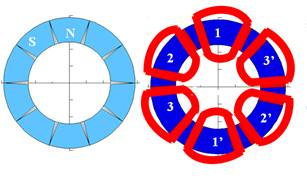Figure 1. PMSM structure.

The 3 D structure of PMSM is illustrated by figure 2.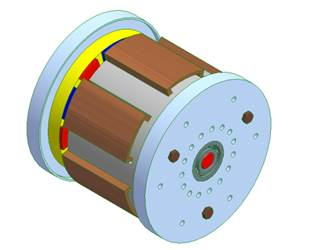Figure 2. 3 D structure of PMSM.

The dimensioning model of this structure is detailed in .

3.1. Assumptions Used for Modeling

We recall firstly our assumptions about thermal modeling of magnetic components to justify the principles of selected measures that lead to the measurement of average temperatures. Temperatures are assumed uniform in the material and in the different coils.

We consider an ambient temperature of 40° C and we assume a maximum permissible temperature rise of 100 ° C for the insulation. This warming-up is determined by the temperature holding of the conductors and the slots bottom insulators. At the same way, the maximum warming-up of the magnets (Sm-Co) must be taken in account and we admit a warming-up of the order of 200°C. In the context of our model, we consider only perfect contacts, and their conduction resistances are low and broken into account in the thermal model of the PMSM , , .

The heat exchange is assumed in the axial direction, because the length of the structure in this direction is much lower than that in the radial direction, in addition heat exchange sections perpendicular to the axis of the motor is much greater than those radials , , .

3.2. Heat transfer in the PMSM

Heat transfer in the PMSM is illustrated by the Figure 3: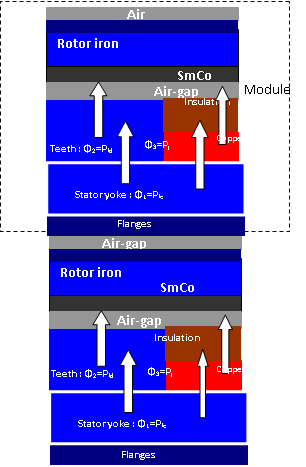Figure 3. The heat transfer in the PMSM.

4. Transient Thermal Model

We selected a model using thermal-electrical analogy. In order to simplify the model, we have the following assumptions:

- Uniform heat generation.

- Uniformity of physical properties across the element.

- Uniformity of the exchanges on each of the faces.

The machine is divided into simple volume elements exchanging heat between them by conduction or convection. Copper losses include slots losses and end winding losses. The transient thermal model of the PMSM structure can then be represented by an analog electrical network, as described in figure 4 , , .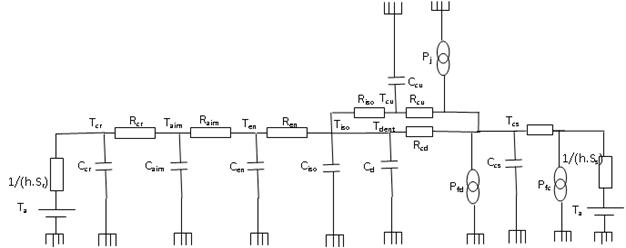Figure 4. Transient thermal model of the PMSM actuator.

Table 1 illustrates the nomenclature of the nodal model diagram:

Table 1. Nomenclature of the nodal model diagram.

 h Convection coefficient Sr Active section of the rotor hf Forced convection coefficient Ss Active section of the stator Rcr Rotor yoke conduction resistance Raim Magnets conduction resistance Ren Air-gap conduction resistance Rcu Copper conduction resistance Rcd Teeth conduction resistance Rcs Stator yoke conduction resistance Pj Copper losses Pfd Teeth iron losses Pfc Stator yoke iron losses Ta Ambient temperature Tcr Rotor yoke temperature Taim Magnets temperature Ten Air-gap temperature Tcu Copper temperature Tiso Average temperature of the insulation Tdent Teeth temperature Tcs Stator yoke temperature Ccr Thermal capacity of the rotor yoke Caim Thermal capacity of magnets Cen Thermal capacity of the air-gap Ccu Thermal capacity of the copper Ciso Thermal capacity of the insulation Cd Thermal capacity of the teeth Ccs Thermal capacity of the stator yoke

For the model presented in figure 4, we define two isothermal zones constituted by the magnetic material on the one hand and by the winding on the other hand.

Previously defined areas are warm seat due to copper losses and iron losses.

Pj, Pfc and Pfd corresponding respectively to the total copper losses in the coils and iron losses of the yoke and stator teeth. The variables Ti correspond to the temperatures at different points of the machine. The terms of thermal resistances are deduced from the resolution of the heat equation border areas.

4.1. Calculation of Conduction Resistances

For the sake of simplification, the conduction along the transverse axis of the stator is not taken although it may be essential, especially in the windings. Heat transfer in a stator element therefore allows one preferred direction, the axial direction. This is reflected by the following heat equation .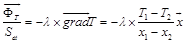(1)

Equation 2 can be derived from a general formula of thermal conduction resistance to an axial flux distribution: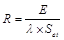(2)

Where λ is the thermal conductivity, Set is the heat exchange section and ΦT is the total heat flux exchanged and E is the thickness of the heat exchange.

The conduction resistances can be deduced from the geometrical equations of PMSM .

For PMSM structures, the conduction resistances of the materials constituting the rotor are expressed by the following relationships:

The conduction resistance of the rotor yoke is expressed by the following relationship: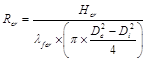(3)

Where λfer is the thermal conductivity of the iron, Hcr is the rotor yoke thikness, De and Di are respectively the exetrnel and internal diameter of the motor.

The conduction resistance of the magnet is expressed by the following relationship: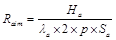(4)

Where λa is thermal conductivity of the magnets, p is the nomber of pole pairs, Sa is the magnet section and Ha is magnet thikness.

The conduction resistance of the air-gap is expressed by the following relationship: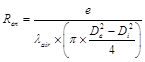(5)

Where λair is the thermal conductivity of air and e is the air-gap thikness.

The conduction resistances respectively of the coils (Rcu), insulation (Riso) of main teeth (Rcd) and stator yoke (Rcs) for the two structures are equal.

The conduction resistance of the coils is expressed by the following relationship: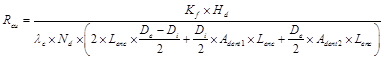(6)

Where λc is the thermal conductivity of copper and Hd is the stator tooth high.

The conduction resistance of the insulator is expressed by the following relationship: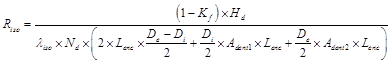(7)

Where λiso is the thermal conductivity of the insulation, Adent1 the lower main tooth angle, Adent2 is higher angle of the main tooth, Nd is the tooth number.

The slot width Lenc is expressed as follows: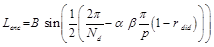(8)

Where rdid is the ratio between the angular width of the inserted tooth and that of the principal tooth. This ratio is optimised by finite elements simulations in order to reduce the flux leakages and to improve the back electromotiv force wave-form.

Where B is equal to: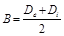(9)

The PMSM configuration is caracterized by variation law of the pole pairs number (p) according to an integer number n varying from one to infinity, the ratio (r) of the number of principal teeth (Nd) by the number of pole pairs, the ratio (n) between the angular width between two principal teeth and that of a principal tooth, the ratio (α) between the angular width of a principal tooth and that of a magnet and the ratio (β) between the angular width of a magnet and the polar step.

The conduction resistance of the main teeth is expressed by the following relationship: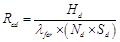(10)

Where Hd is the teeth high, Sd is the main tooth section.

The conduction resistance of the stator yoke is expressed by the following relationship: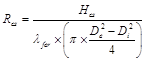(11)

Wher Hcs is the stator yoke thikness.

4.2. Convection Resistances Computation

The convective heat transfer is the preferred mode of transfer within the fluids. Then it is generally much more important than conduction. We must distinguish between natural convection and forced convection.

Density differences related to differences in temperature cause movements of the fluid which is heated in contact with hot body and thus carries the heat to colder areas: the natural convection. The yoke and the outer flanges of the machine in the absence of external fan, undergo this transfer mode. In the internal parts, not brewed areas are rare due to the rotor . The heat exchange coefficient between the housing and the ambient air, can be between 20 and 50 W. K- 1.m -2 for machine natural ventilation and can exceed 80 W. K- 1.m -2 for machines with forced ventilation , .

The only network element that refers to a transfer by convection is Rext , which represents the overall thermal resistance between the surface of the casing, the flanges and the ambient air.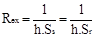(12)

Where h is the heat transfer coefficient, Ss is the area of heat exchange with the stator and Sr is the area of heat exchange with the rotor.

The calculation of external surface of the actuator requires some remarks. Should we consider only the side surfaces (flanges) of the machine or also include the outer surface of the cylinder? Model assumptions, namely a consideration of phenomena only in the axial axis, incite choose the first solution. Wherein the surfaces of heat exchange by convection are expressed by the following relationship: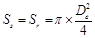(13)

4.3. Calculation of Thermal Capacity

We will study the thermal phenomena of a transient point of view, it is therefore necessary to involve the heat capacities of the materials constituting the components of the machine.

The terms of thermal capacity is calculated from the following relationship between the mass of materials and their massive heat capacity using the following equation , :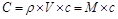(14)

With ρ is the density of the material, V is the volume of the material, c is the mass heat capacity of the material and M is the mass of the material.

4.4. Heat Flux

The heat flux Ф 1 corresponds to iron losses in the stator yoke (Pfc). This flux propagates from the center of gravity of the stator yoke. It is expressed by the following equation :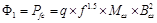(15)

Where q is the quality factor of the metal sheets, Mcs is the mass of the stator yoke and Bcs is the magnetic induction in the stator yoke.

The heat flux Ф2 corresponds to the iron losses in the stator yoke (Pfd). This flux propagates from the center of gravity of the stator teeth. It is expressed by the following equation :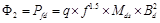(16)

Where Mds is the mass of the stator teeth and Bd is the magnetic induction in the stator teeth.

The heat flux Ф3 corresponds to the copper losses in the stator (Pj). This flux propagates from the center of gravity of the stator copper. It is expressed by the following equation: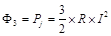(17)

Where R is the resistance of the stator winding, it is expressed by the following relationship: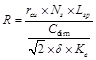(18)

Where rcu is the resistivity of copper, Lsp is the average length of one turn, Ns is the number of phase spires, Cdim is the dimensionnig torque of the motor, δ is the current density in the copper and Ke is the back electromotive force constant.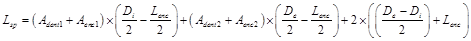(19)

5. Simulation Results

The loss model for PMSM for an electromagnetic switch power chain is located under the environment of Matlab / Simulink 7.1 version. Heat fluxes are calculated based on the inverse modeling approach of the power chain (figure 5).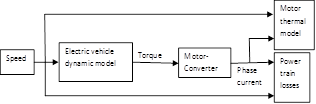Figure 5. Electric vehicle power train model.

Simulation results are obtained for the standard travels (1027 s) during repeated sequences.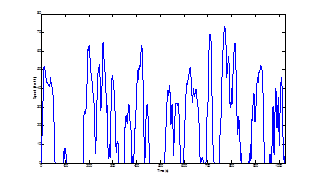Figure 6. Standardized travels.

The simulation of the thermal model of the PMSM structure, with natural convection with air (convection coefficient equal to 30 W/(m².K)), shows the evolution of temperatures in different active parts of the motor (figure 7).Figure 7. Evolution of temperatures in the different active parts of the motor (h = 30 W/(m².K)).

Where Tcr is the average temperature of the rotor yoke, Taim is the average temperature of magnets, Ten is the average temperature of the air gap, Tiso is the average temperature of the insulation, Tcu is the average temperature of the copper, Tdent is the average temperature of the stator teeth and Tcs is the average temperature of the stator yoke.

This figure shows that there's an exceeding of 700 ° C for the the insulation, which proves the need for a cooling system. Several simulations are undertaken for several values ​​ of forced convection coefficient in water, have led to the fixing of the this coefficient to 5000 W/(m².K).

Evolutions of temperature in the different active parts of the engine for operation with a cooling system with forced convection in water h = 5000 W/(m².K) is illustrated in figure 8.Figure 8. Evolution of temperatures in the different active parts of the motor (h = 5000 W/(m².K)).

This figure shows that the temperature of the insulation is reduced to 90 ° C, acceptable value.

6. Conclusion

In this paper we present a methodology for electrothermal modeling of PMSM configuration to define the less powerful and the least expensive cooling system. This approach is based on modeling with nodal method because it provides a fast and acceptable precision for our application. It is compatible to optimization approaches of the design parameters of electric cars. This study led to the selection of a cooling system by water forced convection. Finally, the approach of thermal modeling tool presents a thermal characterization of the PMSM dedicated to the design of these types of actuators. It will be interesting as future work to address the phase of experimental validation of the model presented in this paper.

References

1. M.N. Ajour, L.A. Dissado, J.C. Fothergill, P.N. Norman, "Dielectric spectroscopy of Epoxy/Glass composite materials", Conference on Electrical Insulation and Dielectric Phenomena, (2002), pp.438-441,
2. A. Apicella, L. Egiziano, L. Nicolais and V. Tucci, "Environmental degradation of the electrical and thermal properties of organic insulating materials", Journal of materials science, (1988), vol.23, p. 729-735
3. TOUNSI et R. NEJI: «Design of an Axial Flux Brushless DC Motor with Concentrated Winding for Electric Vehicles», Journal of Electrical Engineering (JEE), Volume 10, 2010 - Edition: 2, pp. 134-146.
4. A. AMMOUS, B. ALLARD, H. MOREL: «Transient temperature mesurements and modeling of IGBT’s under short circuit», IEEE transaction electronic devices, vol. 13, n° 1, 1998, p. 12-25.
5. P. H. Mellor, D. Roberts, D. R Turner. «Lumped Parameter Thermal Model for Electrical machine of TEFC design». IEEE Trans on Electrical Power Application., 1991, 138(5): 205-2018.
6. Gerling D, Dajaku G. Novel «lumped-parameter thermal model for electrical systems» Power Electronics and Applications, 2005 European Conference on. IEEE, 2005: 10 pp.-P. 10.
7. S.TOUNSI, R.NÉJI, F.SELLAMI : « Conception d'un actionneur à aimants permanents pour véhicules électriques »,Revue Internationale de Génie Électrique volume 9/6 2006 - pp.693-718.
8. Staton D, Boglietti A, Cavagnino A. «Solving the more difficult aspects of electric motor thermal analysis in small and medium size industrial induction motors». Energy conversion, IEEE transactions on, 2005, 20(3): 620-628.
9. Sun Xikai, Cheng Ming. «Thermal analysis and cooling system design of dual mechanical port machine for wind power application». IEEE Transactions on Industrial Electronics, May 2013, 60(5): 1724-1733.
10. Zhu Sa, Cheng Ming. «Core loss analysis and calculation of stator permanent magnet machine considering dc-biased magnetic induction». IEEE Transactions on Industrial Electronics. Digital Object Identifier: 10.1109/TIE.2014.2300062.

 Contents 1. 2. 3. 3.1. 3.2. 4. 4.1. 4.2. 4.3. 4.4. 5. 6.
Article ToolsAbstractPDF(302K)